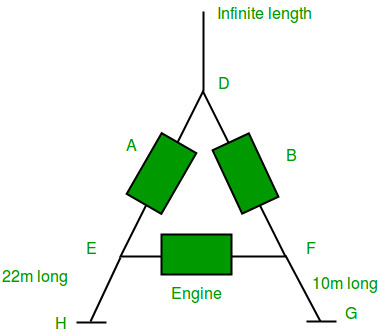Open In App

# Oracle Interview | Set 9 (On campus for Application Development profile)

Online Round:
An online written round was conducted on mettl.com consisting of following sections:
1. General Aptitude – 15 questions

2. Logical Reasoning – 15 questions

3. Technical (All CS subjects) – 20 questions

4. English – 10 questions

Duration was 75 min and 53 members were selected for next round among 200 aspirants.

First Round:
Interviewer introduced himself and asked me the question “Why Oracle?”
He then read my resume and asked me about the projects that I have done and about the things in my resume. Then he asked me to explain algorithm of the project that I’m going to do in the upcoming semester and asked me code it.
He asked me what my favorite subject was. I replied that it was Data Structures. He asked me few simple questions from DS like:
1. Implement queue using linked lists
2. Reverse a string
3. Find the number of palindromes in a big string.
Finally he asked me the following puzzle
He drew this diagram:Engine is of length 12m. A and B are 2 bogies of 10m length each. Engine can turn only in obtuse angles but not in acute angle. For example Engine can turn as FEH but not as FEA. Now, the task is that I have to exchange the positions of A and B with the help of Engine and the engine should remain at the same place.
I tried for 15 minutes but couldn’t solve this problem.

Second Round:
The interviewer went straight into questions. This is the toughest and longest round. I am posed with the following questions:
1. Explain interfaces in Java
2. What is overloading and what is overriding. Explain each with example
3. What are abstract classes in Java and what is the difference between an abstract class and an interface.
4. Find the fifth largest element in a linked list
5. Find the common ancestor of two given nodes in a tree
6. Explain heap sort
7. Find the duplicates in an array
8. An array of elements were given. You have to insert them into BST, Max Heap, B Tree
9. Merge two sorted linked lists
10. Explain three normalizations with example
11. Two SQL queries:
1. Find the maximum salary in emp table
2. Find the name and department of the employee with highest salary
12. Difference between semaphore and mutex
13. PUZZLE: There are 25 horses and only 5 horses can be raced at a time and the top 3 are announced in each such race. What is the minimum number of races required to find the top 3 among 25 horses
14. How is undo operation (ctrl + z) implemented internally?
15. Explain 8 queens algorithm
16. What is time complexity of 8 queens algorithm
I haven’t answered 12, 13 and 16 questions.

Third Round:
In this round I am asked both technical and HR questions.